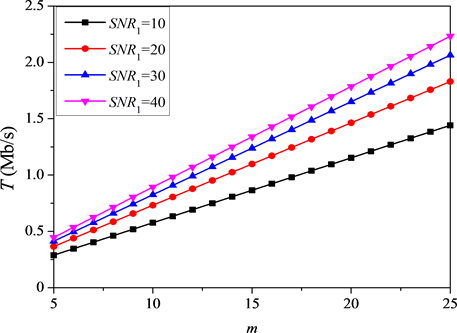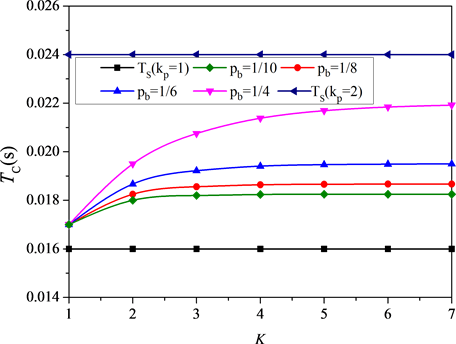﻿ 基于比例公平调度算法的认知无线电系统性能分析

# 基于比例公平调度算法的认知无线电系统性能分析Performance Analysis of Cognitive Radio System Based on Proportional Fair Scheduling Algorithm

Abstract: In order to further improve the utilization of spectrum resources and solve the problem of unscheduled users under the proportional fairness algorithm in the downlink transmission process of LTE systems, this paper proposes to integrate cognitive technologies into the proportional fair scheduling algorithm. Therefore, these unscheduled users have the ability to discover “spectral voids” and make reasonable use of them, thereby effectively utilizing spectrum resources and improving system throughput. Second, because the secondary user (SU) must not only have the cognitive ability but also the reconstruction character, that is how the secondary user should respond when the primary user (PU) occupies the spectrum, continuing to wait or finding a new carrier. In response to this problem, this paper discusses the delay in two cases and gives the decision basis.

1. 引言

2. 比例公平调度算法

${M}^{\ast }=\mathrm{arg}\underset{n=1,2,\cdots ,N}{\mathrm{max}}\frac{{R}_{m,n}\left(t\right)}{{T}_{m,n}\left(t\right)}$ (1)

${R}_{m,n}\left(t\right)=B/N{\mathrm{log}}_{2}\left(1+SNR\right)$ (2)

$SN{R}_{m,n}\left(t\right)=\frac{{S}_{m,n}\left(t\right){H}_{m,n}\left(t\right)}{{N}_{0}B/N}$ (3)

${T}_{m,n}\left(t+1\right)=\left\{\begin{array}{l}\left(1-\frac{1}{{t}_{c}}\right){T}_{m,n}\left(t\right)+\frac{1}{{t}_{c}}{R}_{m,n}\left(t\right)\text{}用户在\text{ }\text{ }t\text{ }\text{ }时刻被调度\\ \left(1-\frac{1}{{t}_{c}}\right){T}_{m,n}\left(t\right)\text{}\text{ }\text{ }用户在\text{ }\text{ }t\text{ }\text{ }时刻未被调度\end{array}$ (4)

3. 加入认知无线电技术的新比例公平调度算法

1) 根据请求的数据速率 ${R}_{m,n}\left(t\right)$ 得到信道质量信息

2) 在每个资源块中找到 ${R}_{m,n}\left(t\right)/{T}_{m,n}\left(t\right)$ 的最大值和它的相应用户

3) 调度这个用户

4) 直到下一个用户在此资源块中满足2)此用户将没有权限被调度

5) 在这些资源块中调度那些用户

6) 认知用户感知频谱空洞

7) 将感知到的自由子载波分配给认知用户

8) 重复这个循环

${C}_{r,k}\left(t\right)=\Delta f{\mathrm{log}}_{2}\left(1+SN{R}_{r,k}\left(t\right)\right)$ (5)

${T}_{r}\left(t\right)=\underset{r}{\sum }\underset{k}{\sum }{\theta }_{r,k}{C}_{r,k}\left(t\right)$ (6)

${\theta }_{r,k}=1$ 表示子载波k被认知用户r占用， ${\theta }_{r,k}=0$ 表示子载波k未被认知用户r占用，同理，用 ${\theta }_{m,n}=1$ 表示第n个资源块被用户m占用， ${\theta }_{m,n}=0$ 表示第n个资源块未被用户m占用。结合公式(1)~(5)加入认知技术后 $t+1$ 时刻系统的吞吐量表示为：

$T\left(t+1\right)=\underset{m}{\sum }\underset{n}{\sum }{\theta }_{m,n}\left[\left(1-\frac{1}{{t}_{c}}\right){T}_{m,n}\left(t\right)+\frac{1}{{t}_{c}}{R}_{m,n}\left(t\right)\right]+\underset{r}{\sum }\underset{k}{\sum }{\theta }_{r,k}{C}_{r,k}\left(t+1\right)$ (7)

$T\left(t+1\right)=\underset{m}{\sum }\underset{n}{\sum }{\theta }_{m,n}\left[\left(1-\frac{1}{{t}_{c}}\right){T}_{m,n}\left(t\right)+\frac{1}{{t}_{c}}{R}_{m,n}\left(t\right)\right]$ (8)

4. 对认知用户的管理(a)(b)

Figure 1. Data transmission diagram

5. 数值分析Figure 2. Relationship between throughput T and number of users m under different $SN{R}_{m,n}$Figure 3. The relationship between system throughput T and r under different mFigure 4. The relationship between back-off times k and TC under different Pb

6. 结束语

 Issariyakul, T., Pillutla, L.S. and Krishnamurthy, V. (2009) Tuning Radio Resource in an Overlay Cognitive Radio Network for TCP: Greed Isn’t Good. IEEE Communications Magazine, 47, 57-63.
https://doi.org/10.1109/MCOM.2009.5183473

 Srinivasa, S. and Jafar, S.A. (2006) The Throughput Potential of Cognitive Radio: A Theoretical Perspective. IEEE Fortieth Asilomar Conference on Signals, Systems and Computers, Pacific Grove, CA, 29 October-1 November 2006, 221-225.

 Shalaby, M., Shokair, M. and Abdo, Y.S.E. (2014) Enhancement of Geometry and Throughput in LTE Femtocells Cognitive Radio Networks. Wireless Personal Communications, 77, 649-659.
https://doi.org/10.1007/s11277-013-1527-8

 Costa, M. and Ephremides, A. (2016) Energy Efficiency versus Performance in Cognitive Wireless Networks. IEEE Journal on Selected Areas in Communications, 34, 1336-1347.
https://doi.org/10.1109/JSAC.2016.2520219

 Esmaeelzadeh, V., Hosseini, E.S., Berangi, R., et al. (2016) Modeling of Rate-Based Congestion Control Schemes in Cognitive Radio Sensor Networks. Ad Hoc Networks, 36, 177-188.